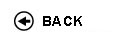Politecnico di Torino
04LSILM, 04LSILJ, 04LSILL, 04LSIOD, 04LSIPC
Mathematical methods
1st degree and Bachelor-level of the Bologna process in Computer Engineering - Torino
1st degree and Bachelor-level of the Bologna process in Telecommunications Engineering - Torino
1st degree and Bachelor-level of the Bologna process in Electronic Engineering - Torino
Espandi...
 Teacher Status SSD Les Ex Lab Tut Years teaching Recupero VincenzoA2 MAT/05 70 30 0 0 1
 SSD CFU Activities Area context MAT/05MAT/06 64 A - Di baseA - Di base Matematica, informatica e statisticaMatematica, informatica e statistica
Esclusioni:
06BQX
 Subject fundamentals The course aims at completing the students' education in basic mathematics, by introducing the theory of analytic functions, distributions, Fourier and Laplace transforms, and discrete and continuous probability. Such topics play an essential role in engineering applications. Examples and motivation will be drawn from problems in electronic and telecommunication engineering and physics, offering further insights. Expected learning outcomes a) Knowledge and understanding Students are taught some basic mathematical notions and tools for solving various problems ranging from signals analysis to the study of random phenomena. The theory of distributions provides a general language which enables to deal with signals arising in impulsive or discontinuous phenomena: this theory is the natural setting for the study of the Fourier and Laplace transforms. Students learn the techniques for the computation of the transforms of the main distributions: delta Dirac, Dirac comb, and piecewise regular functions included. Complex analysis is the proper setting for the theory of the Laplace transform and is the advanced tool for the analysis of singular phenomena and for the computation of integrals. Moreover, students are provided with the main probabilistic tools necessary for solving problems under uncertainty. They learn how to deal with random phenomena and with the variables involved in them. b) Applying knowledge and understanding At the end of the course students will be able to apply the analytical techniques required for the analysis of the signals of any nature (impulsive, erratic, etc.). Also, they will be expected to have acquired the skills to evaluate the probability of outcomes and extrapolate information useful in solving problems in electronic and telecommunication engineering. The ability to apply the gained knowledge will be verified through class exercises. Prerequisites / Assumed knowledge Students are required to be familiar with the notions and tools of the mathematics courses of the first two years: these include differential and integral calculus of one or several variables. Contents 1. (27h) Function theory of complex variable: differentiability, Cauchy-Riemann equations, line integrals. Cauchy theorem, Cauchy integral formula, Taylor series of analytic functions, Laurent series. Residue theorem, computation of residues and application to the calculation of integrals. 2. (15h) Theory of distributions: definitions and basic operations (algebraic operations, translation, rescaling, derivatives), Dirac delta, p.v.(1/t), Dirac comb. Convolution of functions and distributions. 3. (18h) Fourier and Laplace transforms of functions and tempered distributions: definitions and properties, inverse transforms, inversion formula. Notable transforms. 4. (10h) Combinatorics, probability measures and related elementary properties. Conditional probability and independence. 5. (15h) Discrete and continuous random variables, distribution of a random variable. Notable examples. 6. (15h) Expectation, joint distribution, independence correlation, conditional expected values. Delivery modes Exercises will cover the topics of the lectures. Some of them will be carried out by the teacher at the blackboard, others will actively involve the students. Texts, readings, handouts and other learning resources Lecture notes will be available in the course web page. Recommended textbook in probability: Ross, S. 'A first course in probability', Pearson Ed. Int., (8th ed., 2010). Assessment and grading criteria Two hours written exam composed of two parts: 1. Multiple choice test. The necessary condition to pass the exam is to correctly answer to at least 5 out of the 10 questions of the multiple choice test. Any correct answer scores 1 point. 2. Exercises composed of different questions. The score for the exercises is 22 points. Programma definitivo per l'A.A.2015/16© Politecnico di Torino
Corso Duca degli Abruzzi, 24 - 10129 Torino, ITALY